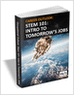MaterialsDesign CenterProcessesUnit ConversionFormulasMathematicsCalculatorsDiscussion ForumTrade PublicationsDirectory Service
Mechanical Engineers Outlook

Guide for those interested in becoming a mechanical engineer. Includes qualifications, pay, and job duties.

CNC Machining Design Guide

STEM Career Outlook

Wages, employment opportunities, and growth projections for STEM jobs.

Learn the best principles to negotiate the salary you deserve!

more free publicationsGlossary » Units » Force » Kilopound Force (kip Force)Kilopound Force (kip Force) (kipf) is a unit in the category of Force. This unit is commonly used in the UK, US unit systems. Kilopound Force (kip Force) (kipf) has a dimension of MLT-2 where M is mass, L is length, and T is time. It can be converted to the corresponding standard SI unit N by multiplying its value by a factor of 4448.22161526.
Note that the seven base dimensions are M (Mass), L (Length), T (Time), Q (Temperature), N (Aamount of Substance), I (Electric Current), and J (Luminous Intensity).

Other units in the category of Force include A.u. of Force (a.u.), Crinal (crinal), Dyne (dyn), Gram Force (gf), Joule Per Centimeter (J/cm), Kilogram Force (kgf, kgp), Kilogram Meter Per Square Second (kg-m/s2), Newton (N), Ounce Force (av.) (ozf (av.)), Pound Force (lbf (av.), lbf), Pound Force Foot Per Inch (lbf-ft/in), Poundal (pdl), Slug Foot Per Square Second (slug-ft/s2), Slug Force, Square Foot Inch of Mercury (0°C) (ft2-inHg (0 °C)), Square Foot Inch of Mercury (15.56°C) (ft2-inHg (15.56 °C)), Ton Force (long), Ton Force (metric), and Ton Force (short).N/ARelated PagesMechanical Engineers Outlook

Guide for those interested in becoming a mechanical engineer. Includes qualifications, pay, and job duties.CNC Machining Design Guide